# Chapter 6 Supervised Learning

In this chapter, we will move beyond statistical regression, and introduce some of the popular machine learning methods.

## 6.1 Read previously saved data

ObsData <- readRDS(file = "data/rhcAnalytic.RDS")
levels(ObsData$Death)=c("No","Yes") out.formula1 <- readRDS(file = "data/form1.RDS") out.formula2 <- readRDS(file = "data/form2.RDS") ## 6.2 Continuous outcome ### 6.2.1 Cross-validation LASSO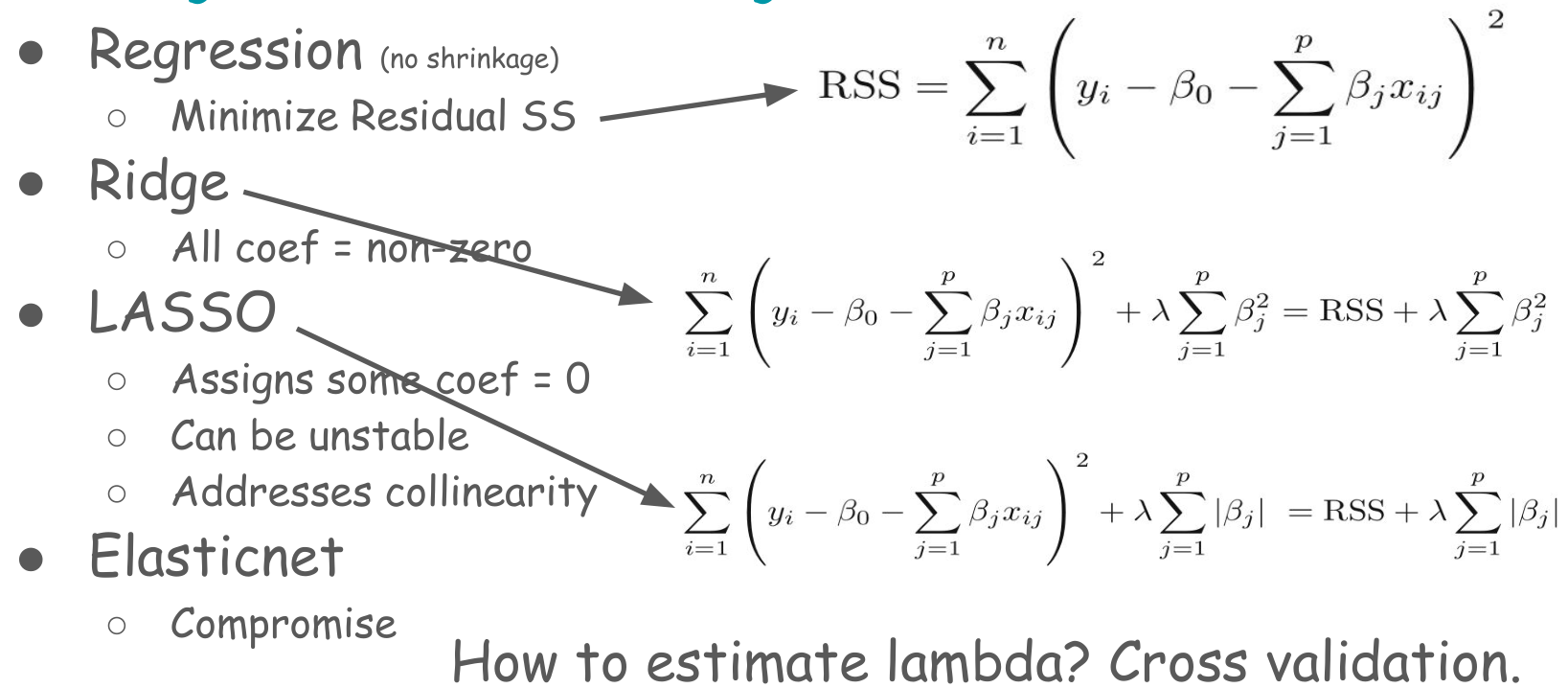ctrl <- trainControl(method = "cv", number = 5) fit.cv.con <- train(out.formula1, trControl = ctrl, data = ObsData, method = "glmnet", lambda= 0, tuneGrid = expand.grid(alpha = 1, lambda = 0), verbose = FALSE) fit.cv.con ## glmnet ## ## 5735 samples ## 50 predictor ## ## No pre-processing ## Resampling: Cross-Validated (5 fold) ## Summary of sample sizes: 4588, 4587, 4587, 4589, 4589 ## Resampling results: ## ## RMSE Rsquared MAE ## 25.14179 0.05746966 15.20167 ## ## Tuning parameter 'alpha' was held constant at a value of 1 ## Tuning ## parameter 'lambda' was held constant at a value of 0 ### 6.2.2 Cross-validation Ridge ctrl <- trainControl(method = "cv", number = 5) fit.cv.con <-train(out.formula1, trControl = ctrl, data = ObsData, method = "glmnet", lambda= 0, tuneGrid = expand.grid(alpha = 0, lambda = 0), verbose = FALSE) fit.cv.con ## glmnet ## ## 5735 samples ## 50 predictor ## ## No pre-processing ## Resampling: Cross-Validated (5 fold) ## Summary of sample sizes: 4588, 4589, 4587, 4588, 4588 ## Resampling results: ## ## RMSE Rsquared MAE ## 25.0993 0.05746023 15.22744 ## ## Tuning parameter 'alpha' was held constant at a value of 0 ## Tuning ## parameter 'lambda' was held constant at a value of 0 ## 6.3 Binary outcome ### 6.3.1 Cross-validation LASSO ctrl<-trainControl(method = "cv", number = 5, classProbs = TRUE, summaryFunction = twoClassSummary) fit.cv.bin<-train(out.formula2, trControl = ctrl, data = ObsData, method = "glmnet", lambda= 0, tuneGrid = expand.grid(alpha = 1, lambda = 0), verbose = FALSE, metric="ROC") fit.cv.bin ## glmnet ## ## 5735 samples ## 50 predictor ## 2 classes: 'No', 'Yes' ## ## No pre-processing ## Resampling: Cross-Validated (5 fold) ## Summary of sample sizes: 4588, 4588, 4589, 4588, 4587 ## Resampling results: ## ## ROC Sens Spec ## 0.7546214 0.4634742 0.8535823 ## ## Tuning parameter 'alpha' was held constant at a value of 1 ## Tuning ## parameter 'lambda' was held constant at a value of 0 • Not okay to select variables from a shrinkage model, and then use them in a regular regression ### 6.3.2 Cross-validation Ridge ctrl<-trainControl(method = "cv", number = 5, classProbs = TRUE, summaryFunction = twoClassSummary) fit.cv.bin<-train(out.formula2, trControl = ctrl, data = ObsData, method = "glmnet", lambda= 0, tuneGrid = expand.grid(alpha = 0, lambda = 0), verbose = FALSE, metric="ROC") fit.cv.bin ## glmnet ## ## 5735 samples ## 50 predictor ## 2 classes: 'No', 'Yes' ## ## No pre-processing ## Resampling: Cross-Validated (5 fold) ## Summary of sample sizes: 4588, 4589, 4588, 4587, 4588 ## Resampling results: ## ## ROC Sens Spec ## 0.7528764 0.4610002 0.8524987 ## ## Tuning parameter 'alpha' was held constant at a value of 0 ## Tuning ## parameter 'lambda' was held constant at a value of 0 ### 6.3.3 Cross-validation Elastic net • Alpha = mixing parameter • Lambda = regularization or tuning parameter • We can use expand.grid for model tuning ctrl<-trainControl(method = "cv", number = 5, classProbs = TRUE, summaryFunction = twoClassSummary) fit.cv.bin<-train(out.formula2, trControl = ctrl, data = ObsData, method = "glmnet", tuneGrid = expand.grid(alpha = seq(0.1,.2,by = 0.05), lambda = seq(0.05,0.3,by = 0.05)), verbose = FALSE, metric="ROC") fit.cv.bin ## glmnet ## ## 5735 samples ## 50 predictor ## 2 classes: 'No', 'Yes' ## ## No pre-processing ## Resampling: Cross-Validated (5 fold) ## Summary of sample sizes: 4587, 4588, 4588, 4588, 4589 ## Resampling results across tuning parameters: ## ## alpha lambda ROC Sens Spec ## 0.10 0.05 0.7530058 0.3695986568 0.8965624 ## 0.10 0.10 0.7493765 0.2742170043 0.9336408 ## 0.10 0.15 0.7445050 0.1748601904 0.9677600 ## 0.10 0.20 0.7388230 0.0894189104 0.9871036 ## 0.10 0.25 0.7322015 0.0253323951 0.9959703 ## 0.10 0.30 0.7254256 0.0019863462 0.9997315 ## 0.15 0.05 0.7519700 0.3537017148 0.9035477 ## 0.15 0.10 0.7453497 0.2200659235 0.9529844 ## 0.15 0.15 0.7356439 0.1023307779 0.9849553 ## 0.15 0.20 0.7247385 0.0178820538 0.9959695 ## 0.15 0.25 0.7170856 0.0004962779 0.9997315 ## 0.15 0.30 0.7107273 0.0000000000 1.0000000 ## 0.20 0.05 0.7507079 0.3328284138 0.9140258 ## 0.20 0.10 0.7394339 0.1693974297 0.9674904 ## 0.20 0.15 0.7242395 0.0372492377 0.9919405 ## 0.20 0.20 0.7140885 0.0014900683 0.9997315 ## 0.20 0.25 0.7069905 0.0000000000 1.0000000 ## 0.20 0.30 0.6971477 0.0000000000 1.0000000 ## ## ROC was used to select the optimal model using the largest value. ## The final values used for the model were alpha = 0.1 and lambda = 0.05. plot(fit.cv.bin)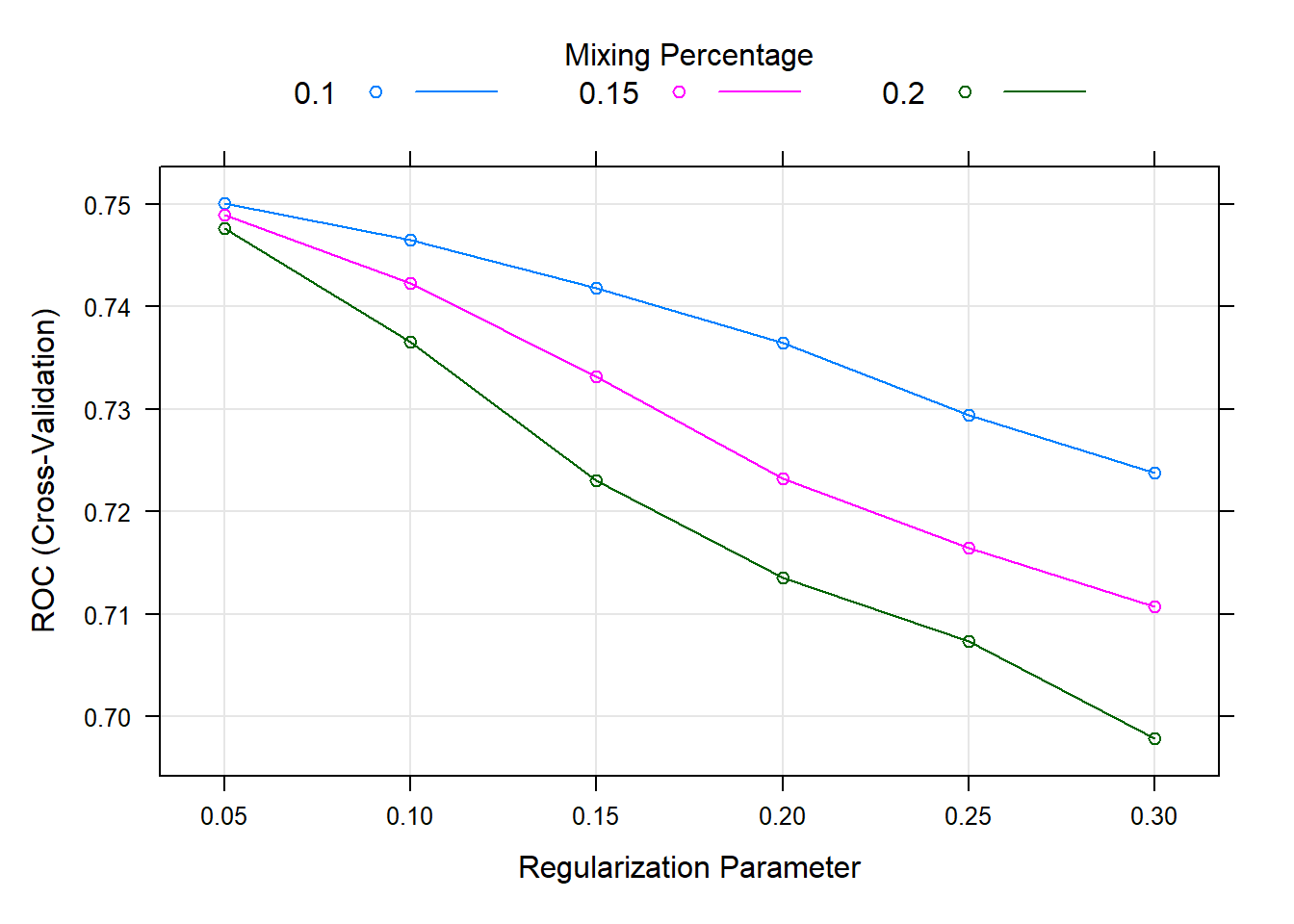### 6.3.4 Decision tree • Decision tree • Referred to as Classification and regression trees or CART • Covers • Classification (categorical outcome) • Regression (continuous outcome) • Flexible to incorporate non-linear effects automatically • No need to specify higher order terms / interactions • Unstable, prone to overfitting, suffers from high variance #### 6.3.4.1 Simple CART require(rpart) summary(ObsData$DASIndex) # Duke Activity Status Index
##    Min. 1st Qu.  Median    Mean 3rd Qu.    Max.
##   11.00   16.06   19.75   20.50   23.43   33.00
cart.fit <- rpart(Death~DASIndex, data = ObsData)
par(mfrow = c(1,1), xpd = NA)
plot(cart.fit)
text(cart.fit, use.n = TRUE)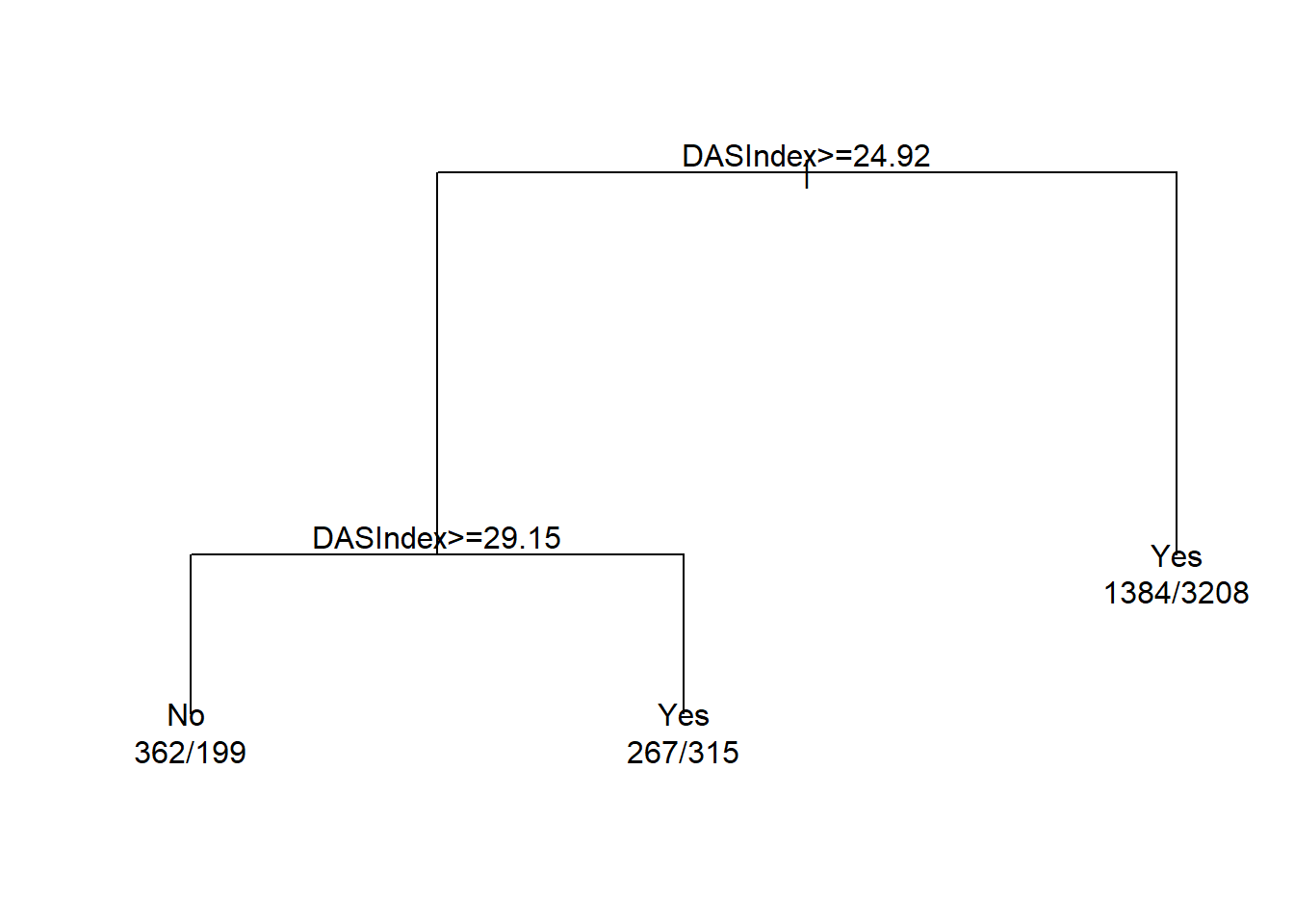print(cart.fit)
## n= 5735
##
## node), split, n, loss, yval, (yprob)
##       * denotes terminal node
##
## 1) root 5735 2013 Yes (0.3510026 0.6489974)
##   2) DASIndex>=24.92383 1143  514 No (0.5503062 0.4496938)
##     4) DASIndex>=29.14648 561  199 No (0.6452763 0.3547237) *
##     5) DASIndex< 29.14648 582  267 Yes (0.4587629 0.5412371) *
##   3) DASIndex< 24.92383 4592 1384 Yes (0.3013937 0.6986063) *
require(rattle)
require(rpart.plot)
require(RColorBrewer)
fancyRpartPlot(cart.fit, caption = NULL)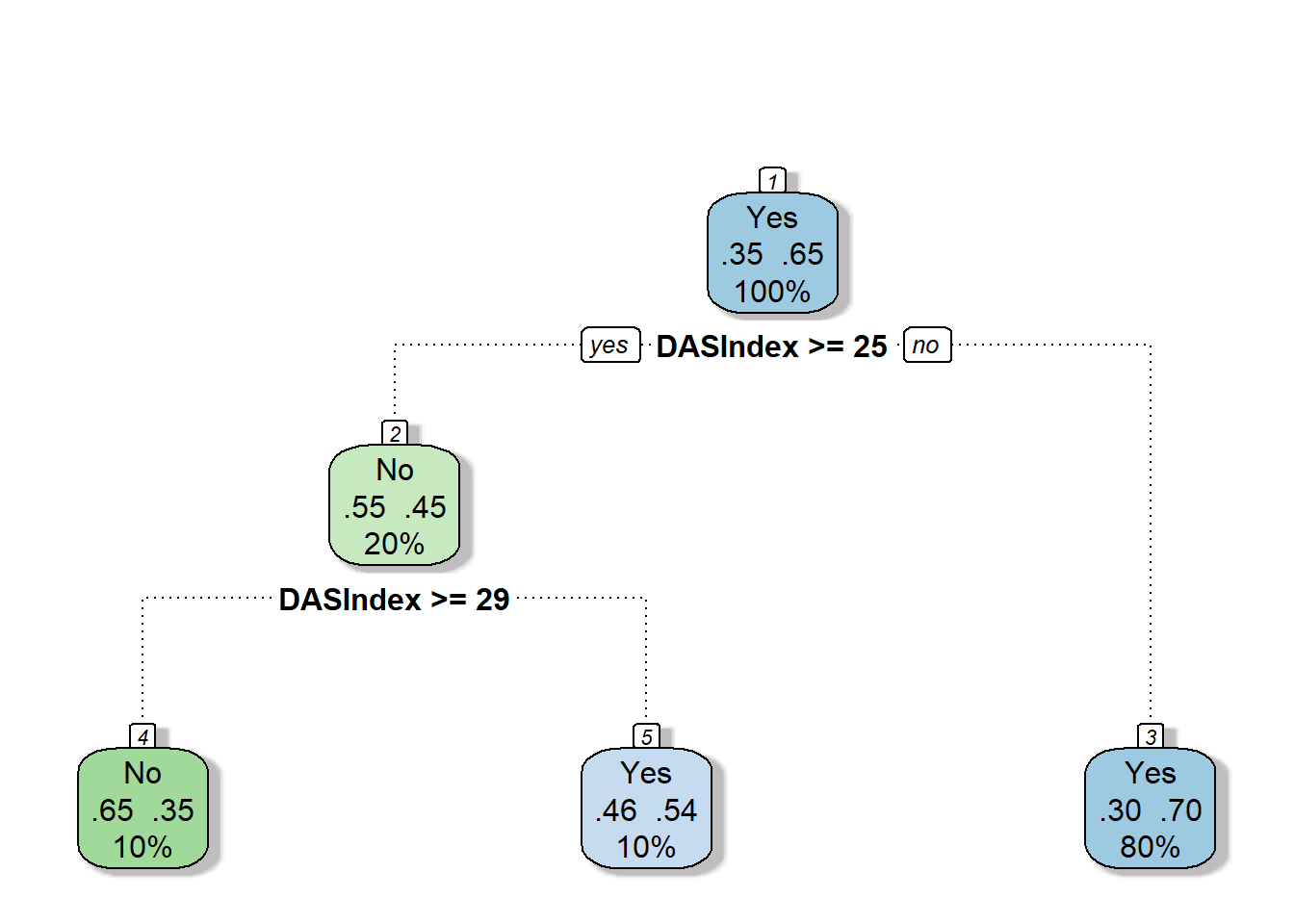##### 6.3.4.1.1 AUC
require(pROC)
obs.y2<-ObsData$Death pred.y2 <- as.numeric(predict(cart.fit, type = "prob")[, 2]) rocobj <- roc(obs.y2, pred.y2) ## Setting levels: control = No, case = Yes ## Setting direction: controls < cases rocobj ## ## Call: ## roc.default(response = obs.y2, predictor = pred.y2) ## ## Data: pred.y2 in 2013 controls (obs.y2 No) < 3722 cases (obs.y2 Yes). ## Area under the curve: 0.5912 plot(rocobj)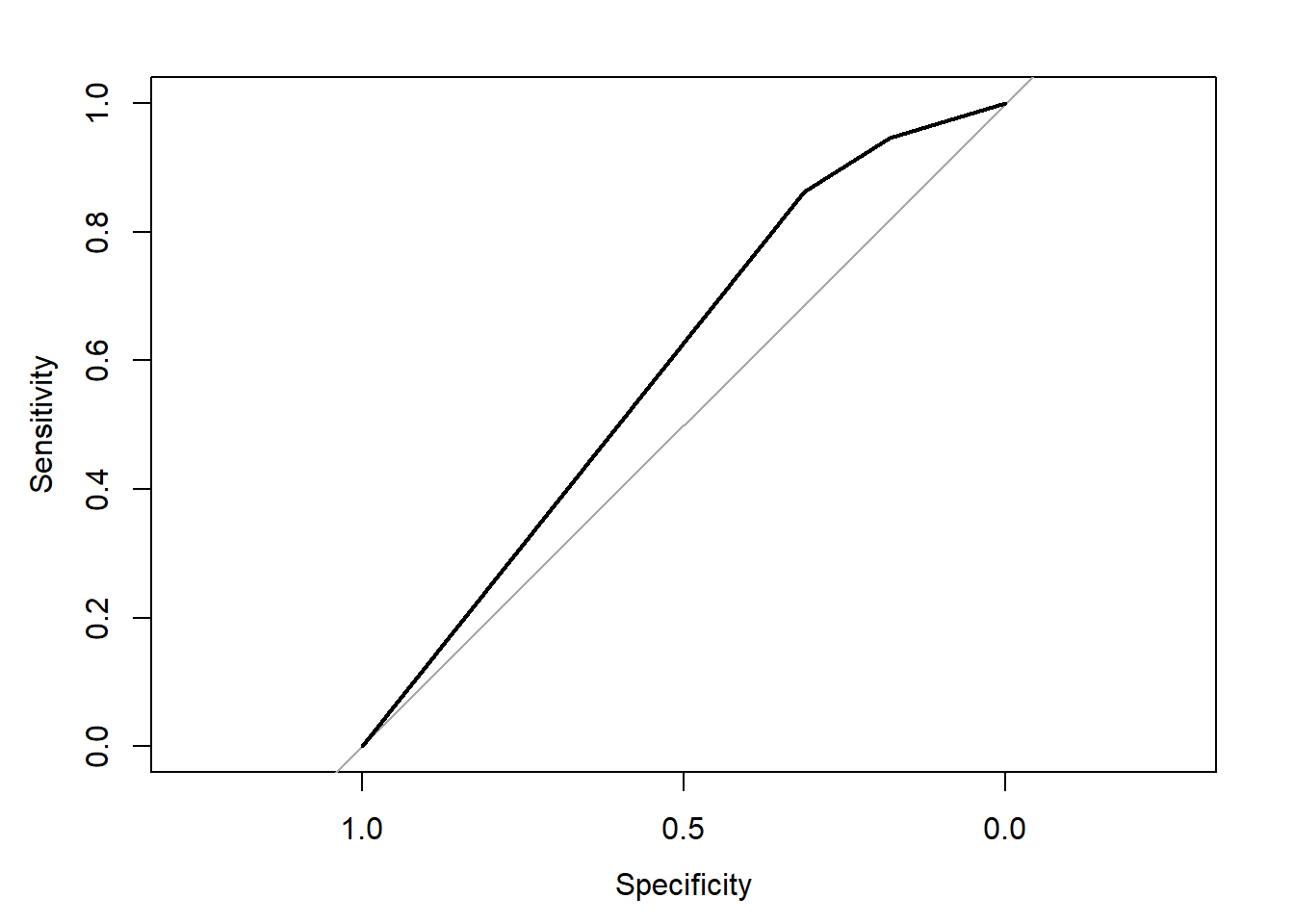auc(rocobj) ## Area under the curve: 0.5912 #### 6.3.4.2 Complex CART More variables out.formula2 ## Death ~ Disease.category + Cancer + Cardiovascular + Congestive.HF + ## Dementia + Psychiatric + Pulmonary + Renal + Hepatic + GI.Bleed + ## Tumor + Immunosupperssion + Transfer.hx + MI + age + sex + ## edu + DASIndex + APACHE.score + Glasgow.Coma.Score + blood.pressure + ## WBC + Heart.rate + Respiratory.rate + Temperature + PaO2vs.FIO2 + ## Albumin + Hematocrit + Bilirubin + Creatinine + Sodium + ## Potassium + PaCo2 + PH + Weight + DNR.status + Medical.insurance + ## Respiratory.Diag + Cardiovascular.Diag + Neurological.Diag + ## Gastrointestinal.Diag + Renal.Diag + Metabolic.Diag + Hematologic.Diag + ## Sepsis.Diag + Trauma.Diag + Orthopedic.Diag + race + income + ## RHC.use require(rpart) cart.fit <- rpart(out.formula2, data = ObsData) ##### 6.3.4.2.1 CART Variable importance cart.fit$variable.importance
##            DASIndex              Cancer               Tumor                 age
##         123.2102455          33.4559400          32.5418433          24.0804860
##   Medical.insurance                 WBC                 edu Cardiovascular.Diag
##          14.5199953           5.6673997           3.7441554           3.6449371
##          Heart.rate      Cardiovascular         Trauma.Diag               PaCo2
##           3.4059248           3.1669125           0.5953098           0.2420672
##           Potassium              Sodium             Albumin
##           0.2420672           0.2420672           0.1984366
##### 6.3.4.2.2 AUC
require(pROC)
obs.y2<-ObsData$Death pred.y2 <- as.numeric(predict(cart.fit, type = "prob")[, 2]) rocobj <- roc(obs.y2, pred.y2) ## Setting levels: control = No, case = Yes ## Setting direction: controls < cases rocobj ## ## Call: ## roc.default(response = obs.y2, predictor = pred.y2) ## ## Data: pred.y2 in 2013 controls (obs.y2 No) < 3722 cases (obs.y2 Yes). ## Area under the curve: 0.5981 plot(rocobj)auc(rocobj) ## Area under the curve: 0.5981 #### 6.3.4.3 Cross-validation CART set.seed(504) require(caret) ctrl<-trainControl(method = "cv", number = 5, classProbs = TRUE, summaryFunction = twoClassSummary) # fit the model with formula = out.formula2 fit.cv.bin<-train(out.formula2, trControl = ctrl, data = ObsData, method = "rpart", metric="ROC") fit.cv.bin ## CART ## ## 5735 samples ## 50 predictor ## 2 classes: 'No', 'Yes' ## ## No pre-processing ## Resampling: Cross-Validated (5 fold) ## Summary of sample sizes: 4587, 4589, 4587, 4589, 4588 ## Resampling results across tuning parameters: ## ## cp ROC Sens Spec ## 0.007203179 0.6304911 0.2816488 0.9086574 ## 0.039741679 0.5725283 0.2488649 0.8981807 ## 0.057128664 0.5380544 0.1287804 0.9473284 ## ## ROC was used to select the optimal model using the largest value. ## The final value used for the model was cp = 0.007203179. # extract results from each test data summary.res <- fit.cv.bin$resample
summary.res
##         ROC      Sens      Spec Resample
## 1 0.6847220 0.3746898 0.8590604    Fold1
## 2 0.6729625 0.2985075 0.8924731    Fold2
## 3 0.6076153 0.2754342 0.9287634    Fold5
## 4 0.5873154 0.2238806 0.9274194    Fold4
## 5 0.5998401 0.2357320 0.9355705    Fold3

## 6.4 Ensemble methods (Type I)

Training same model to different samples (of the same data)

### 6.4.1 Cross-validation bagging

• Bagging or bootstrap aggregation
• independent bootstrap samples (sampling with replacement, B times),
• applies CART on each i (no prunning)
• Average the resulting predictions
• Reduces variance as a result of using bootstrap
set.seed(504)
require(caret)
ctrl<-trainControl(method = "cv", number = 5,
classProbs = TRUE,
summaryFunction = twoClassSummary)
# fit the model with formula = out.formula2
fit.cv.bin<-train(out.formula2, trControl = ctrl,
data = ObsData, method = "bag",
bagControl = bagControl(fit = ldaBag$fit, predict = ldaBag$pred,
aggregate = ldaBag\$aggregate),
metric="ROC")
## Warning: executing %dopar% sequentially: no parallel backend registered
fit.cv.bin
## Bagged Model
##
## 5735 samples
##   50 predictor
##    2 classes: 'No', 'Yes'
##
## No pre-processing
## Resampling: Cross-Validated (5 fold)
## Summary of sample sizes: 4587, 4589, 4587, 4589, 4588
## Resampling results:
##
##   ROC        Sens       Spec
##   0.7506666  0.4485809  0.8602811
##
## Tuning parameter 'vars' was held constant at a value of 63
• bagging improves prediction accuracy
• over prediction using a single tree
• Looses interpretability
• as this is an average of many diagrams now
• But we can get a summary of the importance of each variable

#### 6.4.1.1 Bagging Variable importance

caret::varImp(fit.cv.bin, scale = FALSE)
## ROC curve variable importance
##
##   only 20 most important variables shown (out of 50)
##
##                    Importance
## age                    0.6159
## APACHE.score           0.6140
## DASIndex               0.5962
## Cancer                 0.5878
## Creatinine             0.5835
## Tumor                  0.5807
## blood.pressure         0.5697
## Glasgow.Coma.Score     0.5656
## Disease.category       0.5641
## Temperature            0.5584
## DNR.status             0.5572
## Hematocrit             0.5525
## Weight                 0.5424
## Bilirubin              0.5397
## income                 0.5319
## RHC.use                0.5263
## Dementia               0.5252
## Congestive.HF          0.5250
## Hematologic.Diag       0.5250

### 6.4.2 Cross-validation boosting

• Boosting
• sequentially updated/weighted bootstrap based on previous learning
set.seed(504)
require(caret)
ctrl<-trainControl(method = "cv", number = 5,
classProbs = TRUE,
summaryFunction = twoClassSummary)
# fit the model with formula = out.formula2
fit.cv.bin<-train(out.formula2, trControl = ctrl,
data = ObsData, method = "gbm",
verbose = FALSE,
metric="ROC")
fit.cv.bin
## Stochastic Gradient Boosting
##
## 5735 samples
##   50 predictor
##    2 classes: 'No', 'Yes'
##
## No pre-processing
## Resampling: Cross-Validated (5 fold)
## Summary of sample sizes: 4587, 4589, 4587, 4589, 4588
## Resampling results across tuning parameters:
##
##   interaction.depth  n.trees  ROC        Sens       Spec
##   1                   50      0.7218938  0.2145970  0.9505647
##   1                  100      0.7410292  0.2980581  0.9234228
##   1                  150      0.7483014  0.3487142  0.9030028
##   2                   50      0.7414513  0.2960631  0.9263816
##   2                  100      0.7534264  0.3869684  0.8917212
##   2                  150      0.7575826  0.4187512  0.8777477
##   3                   50      0.7496078  0.3626125  0.9070358
##   3                  100      0.7579645  0.4078244  0.8764076
##   3                  150      0.7637074  0.4445909  0.8702298
##
## Tuning parameter 'shrinkage' was held constant at a value of 0.1
##
## Tuning parameter 'n.minobsinnode' was held constant at a value of 10
## ROC was used to select the optimal model using the largest value.
## The final values used for the model were n.trees = 150, interaction.depth =
##  3, shrinkage = 0.1 and n.minobsinnode = 10.
plot(fit.cv.bin)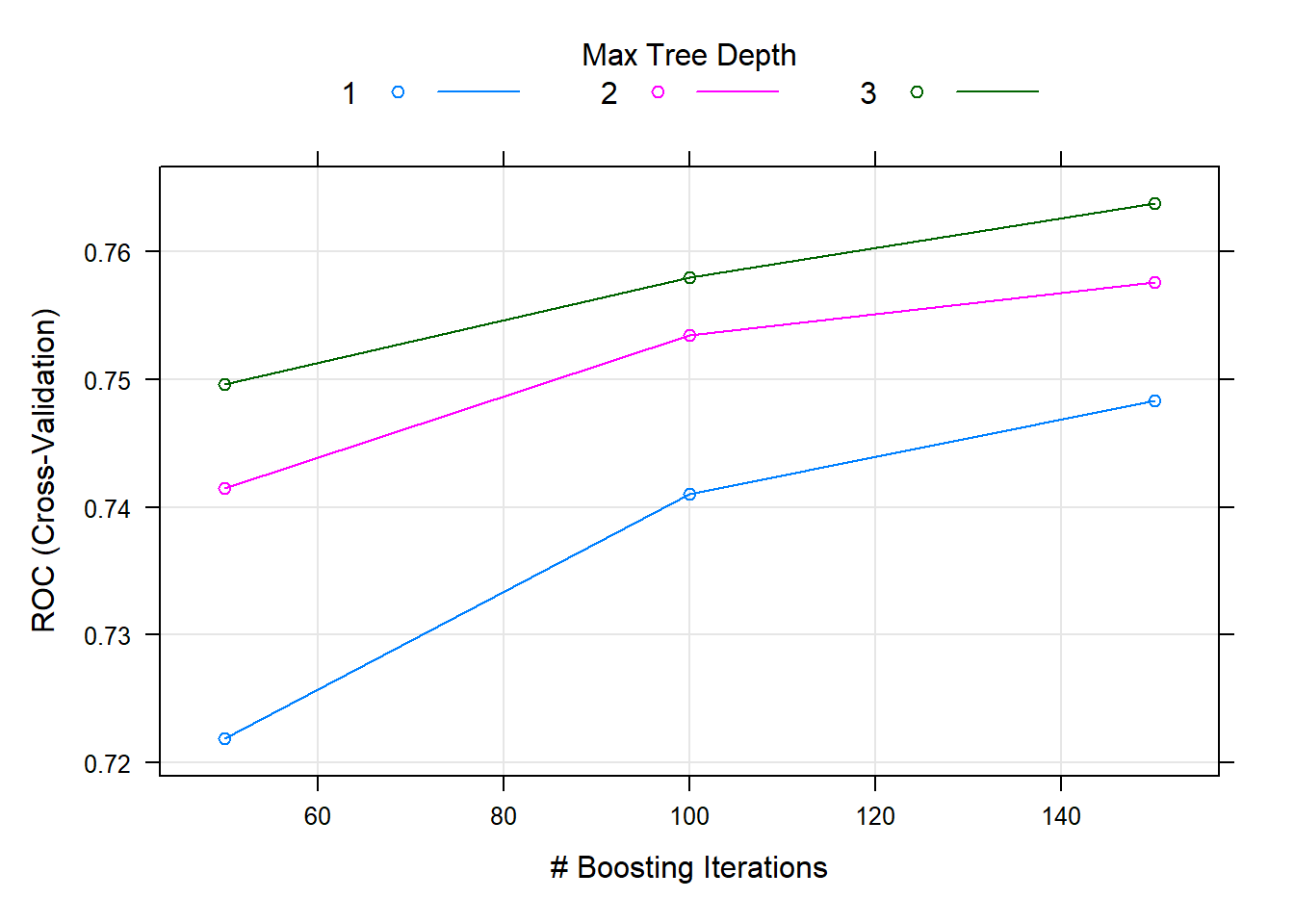## 6.5 Ensemble methods (Type II)

Training different models on the same data

### 6.5.1 Super Learner

• Large number of candidate learners (CL) with different strengths
• Parametric (logistic)
• Non-parametric (CART)
• Cross-validation: CL applied on training data, prediction made on test data
• Final prediction uses a weighted version of all predictions
• Weights = coef of Observed outcome ~ prediction from each CL

### 6.5.2 Steps

Refer to this tutorial for steps and examples!